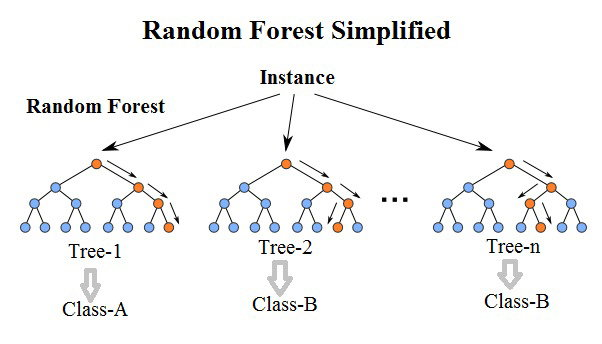02 Aug
02Aug

# Random Forest

Random forests or random decision forests are an ensemble learning method for classification, regression and other tasks, that operate by constructing a multitude of decision trees at training time and outputting the class that is the mode of the classes (classification) or mean prediction (regression) of the individual trees.Random decision forests correct for decision trees' habit of overfitting to their training set. Read moreIt's a supervised classification algorithm. We can see it from its name, which is to create a forest by some way and make it random. There is a direct relationship between the number of trees in the forest and the results it can get: the larger the number of trees, the more accurate the result. But one thing to note is that creating the forest is not the same as constructing the decision with information gain or gain index approach.

Random Forests grows many classification trees. To classify a new object from an input vector, put the input vector down each of the trees in the forest. Each tree gives a classification, and we say the tree "votes" for that class. The forest chooses the classification having the most votes (over all the trees in the forest).

Each tree is grown as follows:

1. If the number of cases in the training set is N, sample N cases at random - but with replacement, from the original data. This sample will be the training set for growing the tree.
2. If there are M input variables, a number m<
3. Each tree is grown to the largest extent possible. There is no pruning.

In the original paper on random forests, it was shown that the forest error rate depends on two things:

• The correlation between any two trees in the forest. Increasing the correlation increases the forest error rate.
• The strength of each individual tree in the forest. A tree with a low error rate is a strong classifier. Increasing the strength of the individual trees decreases the forest error rate.

Reducing m reduces both the correlation and the strength. Increasing it increases both. Somewhere in between is an "optimal" range of m - usually quite wide. Using the oob error rate (see below) a value of m in the range can quickly be found. This is the only adjustable parameter to which random forests is somewhat sensitive.

### Features of Random Forests

• It is unexcelled in accuracy among current algorithms.
• It runs efficiently on large data bases.
• It can handle thousands of input variables without variable deletion.
• It gives estimates of what variables are important in the classification.
• It generates an internal unbiased estimate of the generalization error as the forest building progresses.
• It has an effective method for estimating missing data and maintains accuracy when a large proportion of the data are missing.
• It has methods for balancing error in class population unbalanced data sets.
• Generated forests can be saved for future use on other data.
• Prototypes are computed that give information about the relation between the variables and the classification.
• It computes proximities between pairs of cases that can be used in clustering, locating outliers, or (by scaling) give interesting views of the data.
• The capabilities of the above can be extended to unlabeled data, leading to unsupervised clustering, data views and outlier detection.
• It offers an experimental method for detecting variable interactions.

### How it differs from Decision Tree

The difference between Random Forest algorithm and the decision tree algorithm is that in Random Forest, the process of finding the root node and splitting the feature nodes will run randomly.

#### When to use to decision tree:

• When you want your model to be simple and explainable
• When you want non parametric model
• When you don't want to worry about feature selection or regularization or worry about multi-collinearity.
• You can overfit the tree and build a model if you are sure of validation or test data set is going to be subset of training data set or almost overlapping instead of unexpected.

#### When to use random forest :

• When you don't bother much about interpreting the model but want better accuracy.
• Random forest will reduce variance part of error rather than bias part, so on a given training data set decision tree may be more accurate than a random forest. But on an unexpected validation data set, Random forest always wins in terms of accuracy.

• It's used for classification as well as for regression
• Random Forest algorithm, if there are enough trees in the forest, the classifier won’t overfit the model.
• The classifier of Random Forest can handle missing values [but just not in the same way that CART and other similar decision tree algorithms do]
• The Random Forest classifier can be modeled for categorical values.
• The Random Forest algorithm can be used for identifying the most important features from the training dataset, in other words, feature engineering.Read more

### Random Forest creation pseudocode

• Randomly select “K” features from total “m” features where k << m
• Among the “K” features, calculate the node “d” using the best split point
• Split the node into daughter nodes using the best split
• Repeat the a to c steps until “l” number of nodes has been reached
• Build forest by repeating steps a to d for “n” number times to create “n” number of trees

### Random Forest prediction pseudocode

• Takes the test features and use the rules of each randomly created decision tree to predict the outcome and stores the predicted outcome (target)
• Calculate the votes for each predicted target
• Consider the high voted predicted target as the final prediction from the random forest algorithm